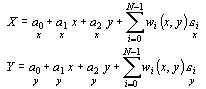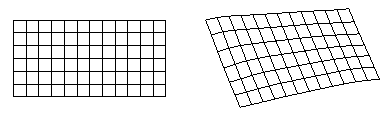# Inverse Distance Squared

The Inverse Distance Squared method is an inverse distance to a power method. The minimum number of control points required for this transformation is four. A RMS value is not reported for this method because this method is a perfect interpolator at the control points. The equation is of the form:The left graphic is the file before using the inverse distance squared transformation. The right graphic is the file after using an inverse distance squared transformation.